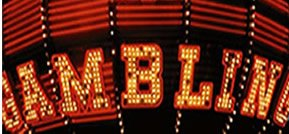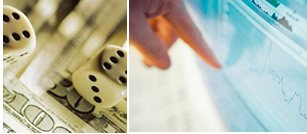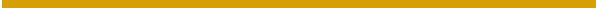GamblingInvestor.com

Ranking Order OF Poker Hands

Royal Flush - Probability 1in 649,740

Straight flush - Probability 1 in 72,193

Four of a kind - Probability 1in 4,165

Full house - Probability 1in 694

Flush - Probability 1in 509

Straight - Probability 1in 255

Three of a kind - Probability 1in 47

Two pair - Probability 1in 21

One pair - Probability 1in 2

No pair  - Probability 1in 2

POKER PROMO